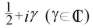Warning! This set of interlinked pages is very much under construction. If you are already familiar with the subject matter, I would appreciate any help you might provide. If you are seeking information on the subject matter, I would be interested to hear what you would ultimately like this page to contain. Thank you.

 Gutzwiller Trace Formula ___  [link]   ___ Selberg Trace Formula | | [link] | | | | [link] | | Riemann's zeta function ___  [link]   ___ Riemann-Weil Explicit Formula | | [link] | | | | [link] | | Connes'(hypothetical) trace formula ___  [link]   ___ (Deninger's) Lefschetz Trace Formulae

"It is easy to understand why Selberg studied trace formulas so intensively: they bear a very striking resemblance to the so-called explicit formulas of prime number theory. Briefly stated, one has:[This is the simplest case of A. Weil's 1952 generalisation of the (number theoretical) explicit formula of Riemann and von Mangoldt. h is a complex-valued function of a real variable which satisfies certain conditions, and g is an integral transform of h. Further notes on this formula can be found here.]

where the nontrivial zeros of the Riemann zeta function are denoted by"

D. Hejhal, The Selberg Trace Formula for PSL(2,R) - Volume I, p. 35

"Selberg noticed this similarity in 1950-51 and was quickly led to a deeper study of trace formulas. Among other things, Selberg found that there is a zeta function which corresponds to [his trace formula] in the same way that [the Riemann zeta function] corresponds to [Weil's explicit formula]. This zeta function is nowadays referred to as the Selberg zeta function; it is usually denoted by Z(s)." [D. Hejhal, Duke Math. J. 1976, p.459]

One possible starting point is the Poisson summation formula. This arises initially as an identity in Fourier analysis, but can be interpreted as a simple 'trace formula' on the torus, as P. Perry has explained. Selberg generalised this, producing a noncommutative analogue of the PSF, which can be interpreted as a trace formula on higher-genus compact Riemannian surfaces. He noted the similarity with a certain form of Weil's explicit formula wherein the nontrivial zeta zeros correspond to eigenvalues. This provides some circumstantial evidence for the Hilbert-Pólya conjecture, and suggests that the explicit formulae of number theory might be trace formulae in some yet-to-be-discovered context.

trace formulae, explicit fomula and number theory page
archive      tutorial      mystery      new      search      home      contact